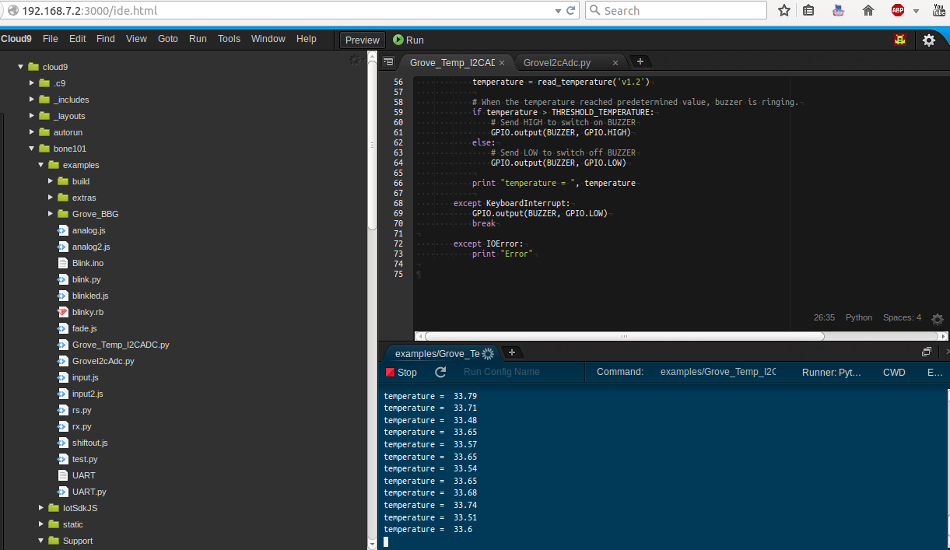# temperature equations with

I have a v1.2 temperature sensor hooked up to a I2C ADC at address 0x50 on my BBG.

From the bash command line on my BBG, I can read the 16-bit word from the conversion result register (#0x00) like this:

```debian@beaglebone:~\$ i2cget -y 1 0x50 0x00 w 0x2104 debian@beaglebone:~\$ i2cget -y 1 0x50 0x00 w 0x2204```

After byte swapping, I interpret these values as 0x0421 and 0x0422 in this example. This is at room temperature in my office, currently around 22 Celsius. If I cover the temperature sensor with my hand, or I put a bottle of ice water close to the sensor, I see the values go up-and-down, anywhere from 0x0300 to 0x0500, and then room temperature settles back around 0x0421, so I suspect it is working correctly.

I’d like to convert these values to Celsius. From the wiki page: seeedstudio.com/wiki/Grove_- … ensor_V1.2 I see the following example:

``` float R = 1023.0/((float)a)-1.0; R = 100000.0*R; float temperature=1.0/(log(R/100000.0)/B+1/298.15)-273.15;```

But plugging in the values I’m seeing, I’m not getting sensible results:

```a = 0x0421; /* aka 1057 decimal */ R = 1023.0 / 1057.0 - 1.0; /* aka -0.032166509 */ temperature=1.0/log(-0.03...) .......cannot take log() of negative number!?```

From the product page github.com/Seeed-Studio/Temperature_Suli where the equation used is:

``` float resistance = (float)(1023.0-val)*10000.0/(float)val; float temp = 1/(log(resistance/10000)/B+1/298.15)-273.15;```

Plugging in the value 0x0421 into this equation, I get:

```a = 0x0421; /* aka 1057 decimal */ resistance = (1023-1057)*10000/1057; /*aka -321 */ temp = 1 / log(-321/10000) ......again, trying to take the log() of a negative number```

Can anyone help?

Hello,

There is an example that comes BBG OS image that implements your requirement. Please refer the same :Thanks and Warm Regards